# Electronics and Communication Engineering - Exam Questions Papers

21.

If the sequence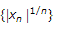converges, then the series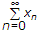converges absolutely is

 A. Ratio test B. Root test C. Divergence test D. Convergence test

Explanation:This is the Root test. Since the signal magnitude is raised to the power of 1/n.

22.

Open loop transfer function of a system isThe phase cross over frequency ωc is

 A.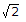B. 1 C. zero D.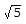Explanation:

GH = - tan- 1 ω - tan- 1 2ω - tan- 1

= - p

tan- 1 ω + tan- 1 2ω = p - tan- 1

tan- l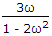= p - tan- 1= - 3ω

23.

The two numbers represent in signed 2's complement form are P = 11101101 and Q = 111001110. If Q subtracted from P, the value obtained in signed 2's complement form is

 A. 100000111 B. 00000111 C. 11111001 D. 111111001

Explanation:

P = 11101101 = - 25

Q = 11100110 = - 18

Thus P - Q = - 25 - (- 18) = - 7

Thus '- 7's two complement representation is 11111001.

24.

The transfer function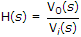of an RLC circuit is given by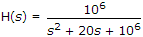quality factor (Q-factor) of this circuit is :

 A. 25 B. 50 C. 100 D. 5000

Explanation: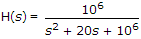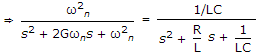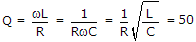.

25.

Consider the circuit shown below of 2 : 1 MUX is given by the function g = ac + bc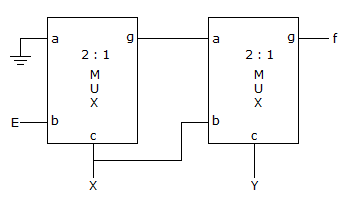Then f is :

 A. X ⊕ Y B. XY + EXY C. XE + XY D. none of these

Explanation:

Since g = ac + bc

i.e. when c = 0, g = b and c = 1, g = a

Now we evaluate

f = (E)(X) + (0)(X) = EX

f = (X)(Y) + (EX)(Y) = XY + EXY.